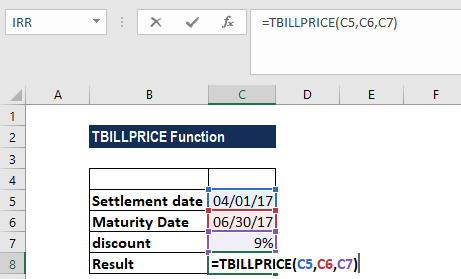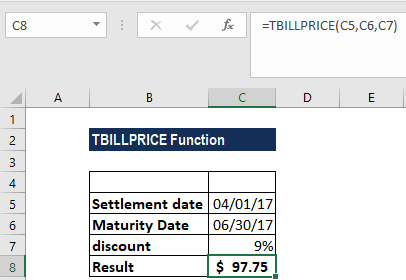# TBILLPRICE Function

Calculates the fair market value of a Treasury bill / bond

## What is the TBILLPRICE Function?

The TBILLPRICE function is categorized under Excel FINANCIAL functions. The function will calculate the fair market value of a Treasury bill / bond.

In financial analysis, TBILLPRICE can be useful in determining the value of a bond and deciding if an investment should be made or not. It helps calculate the FMV of a Treasury bill when we are given the settlement, maturity, and discount rate information.

### Formula

=TBILLPRICE(settlement, maturity, discount)

The TBILLLPRICE function uses the following arguments:

1. Settlement (required argument) – This is the settlement date of the T-bill. The security settlement date is the date after the issue date when the T-bill is traded to the buyer.
2. Maturity (required argument) – This is the T-bill’s maturity date. The maturity date is the date when the T-bill expires.
3. Discount (required argument) – The T-bill’s discount rate.

### How to use the TBILLPRICE Function in Excel?

As a worksheet function, TBILLPRICE can be entered as part of a formula in a cell of a worksheet. To understand the uses of the function, let us consider an example:

#### Example

Suppose we are given the data below:

• Settlement date: 04/01/17
• Maturity date: 06/30/17
• Discount rate: 9%

Using TBILLPRICE function, we can calculate the yield. The formula is:We get the results below:The function returned a value of \$97.75, i.e., a Treasury bill with the above terms would be valued at \$97.75 (rounded to two decimal points).

### A few notes about the TBILLPRICE Function:

1. #NUM! error – Occurs if either:
• The settlement date is greater than or equal to the maturity date
• The maturity date is more than 1 year after the settlement date
• The supplied discount argument is less than or equal to 0.
2. #VALUE! error – Occurs if either:
• Any of the supplied arguments are non-numeric
• The settlement or the maturity arguments are not valid dates.
3. Settlement and maturity are truncated to integers.
4. The settlement and maturity arguments should be supplied to the function as either:
• References to cells containing dates
• Dates returned from other functions or formulas

Thanks for reading CFI’s guide to important Excel functions! By taking the time to learn and master these functions, you’ll significantly improve your financial modeling skills. To learn more, check out these additional CFI resources:

• Excel Functions for Finance